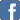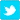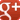# Solving Suko Using Simultaneous Equations

I had to take The Lass and her friend to the pictures last week. I didn't want to watch the film, so I bought myself a Times newspaper and can of Monster "energy drink" (i.e. sticky lemonade) and sat down outside in the sunshine.
On page 4 or so there was a maths puzzle called "Suko", which looked like this:The rules are, you have to put the digits 1 to 9 in the boxes, the total of the digits in the white boxes are 14 etc, and the total of the four digits surrounding each circle is the number in the circle.
------------------------------------------------
As I was in no hurry, I tried doing it by trial and error but hadn't solved it after an hour, so I figured it might be better to use the brute force of simultaneous equations.
So we label the nine boxes A to J (miss out "I" so that you don't mix it up with "1") and get cracking:Clearly, A + B = 14.
A + B + D + E = 20, so D + E = 6.
G + H + D + E = 15, so G + H = 9
H + J + D + E = 16, so H + J = 10.
G + H = 9 and H + J = 10, so G + 1 = J.
Although we don't know what E,F are, we know that B + C + 4 = H + J, so B + C = 6.
A,B are either 9,5 or 8,6, B + C = 6 and you can't use zero, so B can't be bigger than 5.
So B = 5, A = 9 and C = 1.
D + E = 6, and we've used 1 and 5, so D,E are 2,4 (although we don't know which way round at this stage).
That leaves us with the digits 3,6,7,8.
The only pair which adds up to 10 are 3,7 so H,J are 3,7 and F,G are 6,8 (although we don't know which way round at this stage).
We know that G + 1 = J, so the only possible combination is G = 6 and J = 7, so H = 3 and F = 8.
To finish off, A(9) + D - 2 = C(1) + F(8), so D = 2
Doing it this way takes about ten minutes.
------------------------------------------------
Let's pop those numbers back in the grid just to check... ah, sorted: